# Sobolev type inequalities of time-periodic boundary value problems for Heaviside and Thomson cables

## Abstract

We consider a time-periodic boundary value problem of n th order ordinary differential operator which appears typically in Heaviside cable and Thomson cable theory. We calculate the best constant and a family of the best functions for a Sobolev type inequality by using the Green function and apply its results to the cable theory. Physical meaning of a Sobolev type inequality is that we can estimate the square of maximum of the absolute value of AC output voltage from above by the power of input voltage.

MSC:46E35, 41A44, 34B27.

## 1 Introduction

For $n=1,2,3,\dots$ , we consider the following boundary value problem for an n th order ordinary differential operator $P\left(d/dt\right)$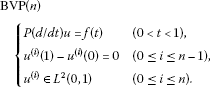(1.1)

The characteristic polynomial with real coefficients

$P\left(z\right)=\prod _{j=0}^{n-1}\left(z+{a}_{j}\right)=\sum _{j=0}^{n}{p}_{j}{z}^{n-j}\phantom{\rule{1em}{0ex}}\left({p}_{0}=1\right)$

is assumed to be a Hurwitz polynomial with real characteristic roots $-{a}_{0},-{a}_{1},\dots ,-{a}_{n-1}$. For the sake of simplicity, we impose the following assumption.

Assumption

${a}_{0}\le {a}_{1}\le \cdots \le {a}_{n-1},\phantom{\rule{1em}{0ex}}{a}_{j}\ne 0\phantom{\rule{0.25em}{0ex}}\left(j=0,1,\dots ,n-1\right).$

We introduce a function $G\left(t\right)$ defined by

$G\left(t\right)=\sum _{k\in \mathbb{Z}}\stackrel{ˆ}{G}\left(k\right)\phi \left(k,t\right),\phantom{\rule{1em}{0ex}}\phi \left(k,t\right)=exp\left(\sqrt{-1}{\omega }_{k}t\right),{\omega }_{k}=2\pi k\phantom{\rule{0.25em}{0ex}}\left(t\in \mathbb{R},k\in \mathbb{Z}\right),$

where

$\stackrel{ˆ}{G}\left(k\right)=\frac{1}{P\left(\sqrt{-1}{\omega }_{k}\right)}\phantom{\rule{1em}{0ex}}\left(k\in \mathbb{Z}\right).$

It is shown later in Section 3 that the function $G\left(t-s\right)$ is the Green function of $BVP\left(n\right)$.

The conclusion of this paper is as follows.

Theorem 1.1 For any function $u\left(t\right)$ satisfying ${u}^{\left(i\right)}\left(t\right)\in {L}^{2}\left(0,1\right)$ ($0\le i\le n$) and ${u}^{\left(i\right)}\left(1\right)-{u}^{\left(i\right)}\left(0\right)=0$ ($0\le i\le n-1$), there exists a positive constant C which is independent of $u\left(t\right)$ such that the following Sobolev type inequality holds:

${\left(\underset{0\le s\le 1}{sup}|u\left(s\right)|\right)}^{2}\le C{\int }_{0}^{1}{|P\left(d/dt\right)u\left(t\right)|}^{2}\phantom{\rule{0.2em}{0ex}}dt.$
(1.2)

Among such C, the best constant $C\left(n;a\right)$ is equal to the square of ${L}^{2}$-norm of the Green function, and it can be expressed as

$C\left(n;a\right)=C\left(n;{a}_{0},\dots ,{a}_{n-1}\right)={\parallel G\parallel }^{2}={\int }_{0}^{1}{|G\left(t\right)|}^{2}\phantom{\rule{0.2em}{0ex}}dt.$

Let $u\left(t\right)=U\left(t\right)$ be a solution of $BVP\left(n\right)$ for $f\left(t\right)=G\left(-t\right)$ ($0). Then, if we replace C by $C\left(n;a\right)$ in (1.2), the equality holds for

$u\left(t\right)=cU\left(t-{t}_{0}\right)\phantom{\rule{1em}{0ex}}\left(0

where ${t}_{0}$ is a real number satisfying $0\le {t}_{0}\le 1$, c is an arbitrary complex number and $U\left(t\right)$ is given by

$U\left(t\right)={\int }_{0}^{1}G\left(t-s\right)G\left(-s\right)\phantom{\rule{0.2em}{0ex}}ds\phantom{\rule{1em}{0ex}}\left(0

The results  and  are prior works related to the subject of the present paper. In  in particular, we considered the boundary value problem of a similar n th linear ordinary differential operator $P\left(d/dt\right)$ and computed the best constant and best function of a Sobolev type inequality by using the Green function. Moreover, the result  relates the best constant of the Sobolev inequality to a 2n th order Hurwitz differential operator. In this paper, we consider a problem similar to that of  with different boundary condition (time-periodic boundary condition).

This paper is organized as follows. First, we construct the Green function using Fourier series expansion in Sections 2 and 3 and derive a Sobolev type inequality from a solution formula to the time-periodic boundary value problem in Section 4. Using the solution formula, we compute the best constant and best function of the Sobolev type inequality as in . The best constant is expressed as a function of ${a}_{0},{a}_{1},\dots ,{a}_{n-1}$. Several concrete forms of the best constant and best function are presented in Section 5. Section 6 presents an interesting application of results for an analog electric circuit. At this point, the physical meaning of a Sobolev type inequality becomes that the square of maximum of the absolute value of AC output voltage is estimated above by the constant multiple of the power of input voltage.

## 2 Fourier series expansion

As a preparation, we explain briefly Fourier series expansion. For $u\left(t\right)\in {L}^{2}\left(0,1\right)$, we have

$u\left(t\right)=\sum _{k\in \mathbb{Z}}\stackrel{ˆ}{u}\left(k\right)\phi \left(k,t\right),$

where the linear transformation ^ is defined by

$u\left(t\right)\stackrel{\stackrel{ˆ}{}}{⟶}\stackrel{ˆ}{u}\left(k\right)={\int }_{0}^{1}u\left(t\right)\overline{\phi }\left(k,t\right)\phantom{\rule{0.2em}{0ex}}dt.$

Note that, for ${u}^{\left(i\right)}\left(t\right)\in {L}^{2}\left(0,1\right)$ ($0\le i\le n$) and ${u}^{\left(i\right)}\left(1\right)-{u}^{\left(i\right)}\left(0\right)=0$ ($0\le i\le n-1$), we have

${u}^{\left(i\right)}\left(t\right)\stackrel{\stackrel{ˆ}{}}{⟶}{\left(\sqrt{-1}{\omega }_{k}\right)}^{i}\stackrel{ˆ}{u}\left(k\right).$

Let us first introduce the trigger function $\left\{t\right\}=t-\left[t\right]$, where $\left[t\right]$ is the integral part of t. Note that $\left\{t\right\}$ is a periodic function of t with period 1. We enumerate important properties of the Fourier transform on ${L}^{2}\left(0,1\right)$.

Proposition 2.1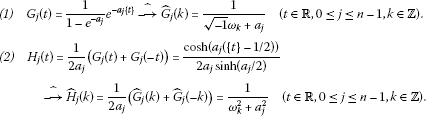Proof of Proposition 2.1 (1) is obtained as follows by a straightforward calculation.

$\begin{array}{rcl}{\stackrel{ˆ}{G}}_{j}\left(k\right)& =& {\int }_{0}^{1}{G}_{j}\left(t\right)\overline{\phi }\left(k,t\right)\phantom{\rule{0.2em}{0ex}}dt={\int }_{0}^{1}\frac{1}{1-{e}^{-{a}_{j}}}{e}^{-\left(\sqrt{-1}{\omega }_{k}+{a}_{j}\right)t}\phantom{\rule{0.2em}{0ex}}dt\\ =& \frac{1}{1-{e}^{-{a}_{j}}}{\left[-\frac{1}{\sqrt{-1}{\omega }_{k}+{a}_{j}}{e}^{-\left(\sqrt{-1}{\omega }_{k}+{a}_{j}\right)t}\right]}_{0}^{1}=\frac{1}{1-{e}^{-{a}_{j}}}\cdot \frac{1-{e}^{-\left(\sqrt{-1}{\omega }_{k}+{a}_{j}\right)}}{\sqrt{-1}{\omega }_{k}+{a}_{j}}\\ =& \frac{1}{\sqrt{-1}{\omega }_{k}+{a}_{j}}.\end{array}$

Next we show (2). Paying attention to $\left\{-t\right\}=-t-\left[-t\right]=1-t$ ($0), we have

$\begin{array}{rcl}{H}_{j}\left(t\right)& =& \frac{1}{2{a}_{j}}\left({G}_{j}\left(t\right)+{G}_{j}\left(-t\right)\right)=\frac{1}{2{a}_{j}}\cdot \frac{1}{1-{e}^{-{a}_{j}}}\left({e}^{-{a}_{j}t}+{e}^{-{a}_{j}\left(1-t\right)}\right)\\ =& \frac{1}{2{a}_{j}}\cdot \frac{1}{{e}^{{a}_{j}/2}-{e}^{-{a}_{j}/2}}\left({e}^{{a}_{j}\left(t-1/2\right)}+{e}^{-{a}_{j}\left(t-1/2\right)}\right)=\frac{cosh\left({a}_{j}\left(t-1/2\right)\right)}{2{a}_{j}sinh\left({a}_{j}/2\right)}.\end{array}$

Using the relation ${\stackrel{ˆ}{G}}_{j}\left(-k\right)=1/\left(-\sqrt{-1}{\omega }_{k}+{a}_{j}\right)$, we have

${\stackrel{ˆ}{H}}_{j}\left(k\right)=\frac{1}{2{a}_{j}}\left({\stackrel{ˆ}{G}}_{j}\left(k\right)+{\stackrel{ˆ}{G}}_{j}\left(-k\right)\right)=\frac{1}{2{a}_{j}}\left(\frac{1}{\sqrt{-1}{\omega }_{k}+{a}_{j}}+\frac{1}{-\sqrt{-1}{\omega }_{k}+{a}_{j}}\right)=\frac{1}{{\omega }_{k}^{2}+{a}_{j}^{2}}.$

This completes the proof of Proposition 2.1. □

## 3 Green function

In this section, we will obtain a concrete expression of the Green function $G\left(t\right)$. Concerning the uniqueness and existence of the solution to $BVP\left(n\right)$, we have the following theorem.

Theorem 3.1 For any function $f\left(t\right)\in {L}^{2}\left(0,1\right)$, $BVP\left(n\right)$ has a unique solution $u\left(t\right)$ expressed as

$u\left(t\right)={\int }_{0}^{1}G\left(t-s\right)f\left(s\right)\phantom{\rule{0.2em}{0ex}}ds\phantom{\rule{1em}{0ex}}\left(0
(3.1)

By using the functions

${G}_{j}\left(t\right)=\frac{1}{1-{e}^{-{a}_{j}}}{e}^{-{a}_{j}\left\{t\right\}}\phantom{\rule{1em}{0ex}}\left(t\in \mathbb{R},0\le j\le n-1\right),$

the Green function $G\left(t\right)$ can be expressed as

$G\left(t\right)=\left({G}_{0}\ast \cdots \ast {G}_{n-1}\right)\left(t\right)\phantom{\rule{1em}{0ex}}\left(t\in \mathbb{R}\right).$
(3.2)

If all ${a}_{j}$’s are distinct, $G\left(t\right)$ is also rewritten as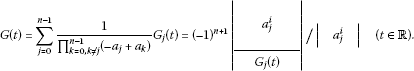(3.3)

In the above determinants, the exponent i and the index j are such that $0\le i\le n-2$ and $0\le j\le n-1$ in the numerator, and $0\le i$, $j\le n-1$ in the denominator.

Remark 3.1 In the case of multiple roots, we can also have the Green function by taking a suitable limit in (3.3). For example, in the case $n=2$, we have

$G\left(t,s\right)=G\left(t-s\right)=\left\{\begin{array}{cc}\frac{{e}^{{a}_{0}\left(1-\left(t-s\right)\right)}}{{\left({e}^{{a}_{0}}-1\right)}^{2}}\left(1+\left({e}^{{a}_{0}}-1\right)\left(t-s\right)\right)\hfill & \left(0

by taking the limit ${a}_{1}\to {a}_{0}$ in (3.3).

In order to prove Theorem 3.1, we transform the expansion of $1/P\left(z\right)$ to a partial fraction. For the partial fraction expansion,

$\frac{1}{P\left(z\right)}=\sum _{j=0}^{n-1}{b}_{j}{\left(z+{a}_{j}\right)}^{-1},\phantom{\rule{1em}{0ex}}{b}_{j}=\frac{1}{{P}^{\prime }\left(-{a}_{j}\right)}=\frac{1}{{\prod }_{k=0,k\ne j}^{n-1}\left(-{a}_{j}+{a}_{k}\right)},$

using the well-known facts (see reference  p.120 (18))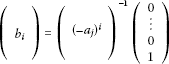and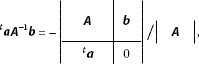where A is any $n×n$ regular matrix, a and b are $n×1$ matrices, we have the following partial fraction expansion: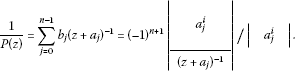(3.4)

The above method is a well-known technique of Heaviside calculus (see reference  A.§5, 22.2, for example).

Proof of Theorem 3.1 Taking the Fourier series expansion on both sides of (1.1), we have

$\sum _{k\in \mathbb{Z}}\stackrel{ˆ}{f}\left(k\right)\phi \left(k,t\right)=f\left(t\right)=P\left(d/dt\right)u\left(t\right)=\sum _{k\in \mathbb{Z}}P\left(\sqrt{-1}{\omega }_{k}\right)\stackrel{ˆ}{u}\left(k\right)\phi \left(k,t\right),$

and hence

$\stackrel{ˆ}{u}\left(k\right)=\stackrel{ˆ}{G}\left(k\right)\stackrel{ˆ}{f}\left(k\right),\phantom{\rule{1em}{0ex}}\stackrel{ˆ}{G}\left(k\right)=\frac{1}{P\left(\sqrt{-1}{\omega }_{k}\right)}=\sum _{j=0}^{n-1}{b}_{j}{\stackrel{ˆ}{G}}_{j}\left(k\right)\phantom{\rule{0.25em}{0ex}}\left(k\in \mathbb{Z}\right),$

where ${\stackrel{ˆ}{G}}_{j}\left(k\right)=1/\left(\sqrt{-1}{\omega }_{k}+{a}_{j}\right)$ ($0\le j\le n-1$). The one and only one solution of BVP is given by

$u\left(t\right)={\int }_{0}^{1}G\left(t,s\right)f\left(s\right)\phantom{\rule{0.2em}{0ex}}ds={\int }_{0}^{1}G\left(t-s\right)f\left(s\right)\phantom{\rule{0.2em}{0ex}}ds\phantom{\rule{1em}{0ex}}\left(0

where $G\left(t,s\right)=G\left(t-s\right)$ is the Green function. For $\stackrel{ˆ}{G}\left(k\right)$, using Proposition 2.1(1) and (3.4), we have (1). (2) follows immediately from

$\stackrel{ˆ}{G}\left(k\right)=\prod _{j=0}^{n-1}{\stackrel{ˆ}{G}}_{j}\left(k\right)\phantom{\rule{1em}{0ex}}\left(k\in \mathbb{Z}\right),$

which completes the proof of Theorem 3.1. □

## 4 Sobolev type inequality

In this section, we give a proof of Theorem 1.1.

Proof of Theorem 1.1 For any function $u\left(t\right)$ satisfying ${u}^{\left(i\right)}\left(t\right)\in {L}^{2}\left(0,1\right)$ ($0\le i\le n$) and ${u}^{\left(i\right)}\left(1\right)-{u}^{\left(i\right)}\left(0\right)=0$ ($0\le i\le n-1$), we define $f\left(t\right)\in {L}^{2}\left(0,1\right)$ by the following relation:

$f\left(t\right)=P\left(d/dt\right)u\left(t\right)\phantom{\rule{1em}{0ex}}\left(0

The inequality

${|u\left(s\right)|}^{2}\le {\int }_{0}^{1}{|G\left(s-t\right)|}^{2}\phantom{\rule{0.2em}{0ex}}dt{\int }_{0}^{1}{|f\left(t\right)|}^{2}\phantom{\rule{0.2em}{0ex}}dt$
(4.1)

is obtained by applying the Schwarz inequality to the solution formula (3.1). The following equality holds from the periodicity of the Green function:

${\int }_{0}^{1}{|G\left(s-t\right)|}^{2}\phantom{\rule{0.2em}{0ex}}dt={\int }_{0}^{1}{|G\left(t\right)|}^{2}\phantom{\rule{0.2em}{0ex}}dt={\parallel G\parallel }^{2}.$

Hence the right-hand side of (4.1) does not depend on s, so we have the following Sobolev type inequality:

${\left(\underset{0\le s\le 1}{sup}|u\left(s\right)|\right)}^{2}\le {\parallel G\parallel }^{2}{\int }_{0}^{1}{|f\left(t\right)|}^{2}\phantom{\rule{0.2em}{0ex}}dt={\parallel G\parallel }^{2}{\int }_{0}^{1}{|P\left(d/dt\right)u\left(t\right)|}^{2}\phantom{\rule{0.2em}{0ex}}dt.$

Taking a solution $u\left(t\right)=U\left(t\right)$ of $BVP\left(n\right)$ for a particular function $f\left(t\right)=G\left(-t\right)$ ($0), we have the following relation:

$U\left(s\right)={\int }_{0}^{1}G\left(s-t\right)G\left(-t\right)\phantom{\rule{0.2em}{0ex}}dt\phantom{\rule{1em}{0ex}}\left(0
(4.2)

In particular, we have

$U\left(0\right)={\int }_{0}^{1}{|G\left(-t\right)|}^{2}\phantom{\rule{0.2em}{0ex}}dt={\parallel G\parallel }^{2}$
(4.3)

by putting $s=0$ in (4.2). We also have

$\begin{array}{rcl}{\parallel G\parallel }^{4}& =& {\left(U\left(0\right)\right)}^{2}\le {\left(\underset{0\le s\le 1}{sup}|U\left(s\right)|\right)}^{2}\le {\parallel G\parallel }^{2}{\int }_{0}^{1}{|P\left(d/dt\right)U\left(t\right)|}^{2}\phantom{\rule{0.2em}{0ex}}dt\\ =& {\parallel G\parallel }^{2}{\int }_{0}^{1}{|G\left(-t\right)|}^{2}\phantom{\rule{0.2em}{0ex}}dt={\parallel G\parallel }^{4}\end{array}$

from (4.2) and (4.3). This means

${\left(\underset{0\le s\le 1}{sup}|U\left(s\right)|\right)}^{2}={\parallel G\parallel }^{2}{\int }_{0}^{1}{|P\left(d/dt\right)U\left(t\right)|}^{2}\phantom{\rule{0.2em}{0ex}}dt,$

which completes the proof of Theorem 1.1. □

## 5 The best constant and best function

The concrete form of the best function $U\left(t\right)$ which appeared in Theorem 1.1, is given by the following lemma.

Lemma 5.1 The best function

$U\left(t\right)={\int }_{0}^{1}G\left(t-s\right)G\left(-s\right)\phantom{\rule{0.2em}{0ex}}ds\phantom{\rule{1em}{0ex}}\left(0
(5.1)

is expressed as

$U\left(t\right)=\left({H}_{0}\ast \cdots \ast {H}_{n-1}\right)\left(t\right)\phantom{\rule{1em}{0ex}}\left(0
(5.2)

If all ${a}_{j}$’s are distinct, $U\left(t\right)$ is also rewritten as

$\begin{array}{rcl}U\left(t\right)& =& \sum _{j=0}^{n-1}\frac{1}{{\prod }_{k=0,k\ne j}^{n-1}\left(-{a}_{j}^{2}+{a}_{k}^{2}\right)}{H}_{j}\left(t\right)\\ =& {\left(-1\right)}^{n+1}|\begin{array}{c}{a}_{j}^{2i}\\ \phantom{\rule{1em}{0ex}}{H}_{j}\left(t\right)\phantom{\rule{1em}{0ex}}\end{array}|/|\phantom{\rule{1em}{0ex}}{a}_{j}^{2i}\phantom{\rule{1em}{0ex}}|\phantom{\rule{1em}{0ex}}\left(0
(5.3)

Here ${H}_{j}\left(t\right)$ is defined as

${H}_{j}\left(t\right)=\frac{cosh\left({a}_{j}\left(\left\{t\right\}-1/2\right)\right)}{2{a}_{j}sinh\left({a}_{j}/2\right)}\phantom{\rule{1em}{0ex}}\left(t\in \mathbb{R},0\le j\le n-1\right).$

In the above determinants, the exponent i and the index j are such that $0\le i\le n-2$ and $0\le j\le n-1$ in the numerator, and $0\le i,j\le n-1$ in the denominator.

Proof of Lemma 5.1 We first prove (5.2). Taking the Fourier series expansion on both sides to the best function, we have

$\begin{array}{rcl}\sum _{k\in \mathbb{Z}}\stackrel{ˆ}{U}\left(k\right)\phi \left(k,t\right)& =& U\left(t\right)={\int }_{0}^{1}G\left(t-s\right)G\left(-s\right)\phantom{\rule{0.2em}{0ex}}ds\\ =& {\int }_{0}^{1}\sum _{k\in \mathbb{Z}}\stackrel{ˆ}{G}\left(k\right)\phi \left(k,t-s\right)\sum _{l\in \mathbb{Z}}\stackrel{ˆ}{G}\left(l\right)\phi \left(l,-s\right)\phantom{\rule{0.2em}{0ex}}ds\\ =& \sum _{k,l\in \mathbb{Z}}\stackrel{ˆ}{G}\left(k\right)\stackrel{ˆ}{G}\left(l\right){\int }_{0}^{1}\phi \left(k,t-s\right)\phi \left(l,-s\right)\phantom{\rule{0.2em}{0ex}}ds\\ =& \sum _{k,l\in \mathbb{Z}}\stackrel{ˆ}{G}\left(k\right)\stackrel{ˆ}{G}\left(l\right)\phi \left(k,t\right){\delta }_{k,-l}\\ =& \sum _{k\in \mathbb{Z}}\stackrel{ˆ}{G}\left(k\right)\stackrel{ˆ}{G}\left(-k\right)\phi \left(k,t\right).\end{array}$

Here we introduce a new function

$R\left(z\right)=\prod _{k=0}^{n-1}\left(z+{a}_{k}^{2}\right).$

Using this expression, we have

$\begin{array}{rcl}\stackrel{ˆ}{U}\left(k\right)& =& \stackrel{ˆ}{G}\left(k\right)\stackrel{ˆ}{G}\left(-k\right)=\prod _{j=0}^{n-1}\frac{1}{{\omega }_{k}^{2}+{a}_{j}^{2}}=\frac{1}{R\left({\omega }_{k}^{2}\right)}=\sum _{j=0}^{n-1}\frac{1}{{R}^{\prime }\left(-{a}_{j}^{2}\right)}{\stackrel{ˆ}{H}}_{j}\left(k\right)\\ =& \sum _{j=0}^{n-1}\frac{1}{{\prod }_{k=0,k\ne j}^{n-1}\left(-{a}_{j}^{2}+{a}_{k}^{2}\right)}{\stackrel{ˆ}{H}}_{j}\left(k\right).\end{array}$

Using (3.4) and Proposition 2.1(2), we have (5.3). Since

$\stackrel{ˆ}{U}\left(k\right)=\frac{1}{R\left({\omega }_{k}^{2}\right)}=\prod _{j=0}^{n-1}{\stackrel{ˆ}{H}}_{j}\left(k\right),$

we have (5.2). This completes the proof of Lemma 5.1. □

Theorem 5.1 For $n=1,2,3,\dots$, the best constant $C\left(n;a\right)$ is expressed by the following concrete forms:

$C\left(1;{a}_{0}\right)=\frac{1}{2{a}_{0}tanh\left({a}_{0}/2\right)}.$

For $n\ge 2$, we have the following expression:

$\begin{array}{rcl}C\left(n;a\right)& =& \frac{1}{2}\sum _{j=0}^{n-1}\frac{1}{{a}_{j}tanh\frac{{a}_{j}}{2}{\prod }_{k=0,k\ne j}^{n-1}\left(-{a}_{j}^{2}+{a}_{k}^{2}\right)}\\ =& \frac{{\left(-1\right)}^{n+1}}{2{a}_{0}\cdots {a}_{n-1}}|\begin{array}{c}{a}_{j}^{2i+1}\\ {\left(tanh\left({a}_{j}/2\right)\right)}^{-1}\end{array}|/|\phantom{\rule{1em}{0ex}}{a}_{j}^{2i}\phantom{\rule{1em}{0ex}}|.\end{array}$

In the above determinants, the exponent i and the index j are such that $0\le i\le n-2$ and $0\le j\le n-1$ in the numerator, and $0\le i,j\le n-1$ in the denominator.

Proof of Theorem 5.1 Putting $t=0$ into (5.1), we have

$U\left(0\right)={\int }_{0}^{1}{|G\left(-s\right)|}^{2}\phantom{\rule{0.2em}{0ex}}ds={\parallel G\parallel }^{2}.$

Using this relation, we are easily able to calculate the best constant of the Sobolev type inequality as follows. If $n=1$, we have

$C\left(1;{a}_{0}\right)={\parallel G\parallel }^{2}=U\left(0\right)={H}_{0}\left(0\right)=\frac{1}{2{a}_{0}tanh\left({a}_{0}/2\right)}.$

If $n\ge 2$, we have from Lemma 5.1,

$\begin{array}{rcl}C\left(n;a\right)& =& {\parallel G\parallel }^{2}=U\left(0\right)=\sum _{j=0}^{n-1}\frac{1}{{\prod }_{k=0,k\ne j}^{n-1}\left(-{a}_{j}^{2}+{a}_{k}^{2}\right)}{H}_{j}\left(0\right)\\ =& \frac{1}{2}\sum _{j=0}^{n-1}\frac{1}{{a}_{j}tanh\frac{{a}_{j}}{2}{\prod }_{k=0,k\ne j}^{n-1}\left(-{a}_{j}^{2}+{a}_{k}^{2}\right)}.\end{array}$

This is also rewritten as

$\begin{array}{rcl}C\left(n;a\right)={\parallel G\parallel }^{2}& =& U\left(0\right)={\left(-1\right)}^{n+1}|\begin{array}{c}{a}_{j}^{2i}\\ {H}_{j}\left(0\right)\end{array}|/|\phantom{\rule{1em}{0ex}}{a}_{j}^{2i}\phantom{\rule{1em}{0ex}}|\\ =& {\left(-1\right)}^{n+1}|\begin{array}{c}{a}_{j}^{2i}\\ {\left(2{a}_{j}tanh\left({a}_{j}/2\right)\right)}^{-1}\end{array}|/|\phantom{\rule{1em}{0ex}}{a}_{j}^{2i}\phantom{\rule{1em}{0ex}}|\\ =& \frac{{\left(-1\right)}^{n+1}}{2{a}_{0}\cdots {a}_{n-1}}|\begin{array}{c}{a}_{j}^{2i+1}\\ {\left(tanh\left({a}_{j}/2\right)\right)}^{-1}\end{array}|/|\phantom{\rule{1em}{0ex}}{a}_{j}^{2i}\phantom{\rule{1em}{0ex}}|.\end{array}$

□

## 6 Heaviside cable and Thomson cable

This section presents an interesting application of the results obtained in the previous sections to engineering fields. We consider the following n-cascaded LRCG units (Figure 1).

${L}_{i}$, ${R}_{i}$, ${C}_{i}$, ${G}_{i}$ are inductance, resistance, capacitance and conductance respectively. They are nonnegative constants and not all of them are zero. ${u}_{i-1}={u}_{i-1}\left(t\right)$ and ${u}_{i}={u}_{i}\left(t\right)$ are input and output voltage respectively. ${v}_{i}={v}_{i}\left(t\right)$ is current. Output end is open, ${v}_{n+1}=0$. Input voltage ${u}_{0}\left(t\right)$ is a given function of t. We investigate the relation between output voltage $u\left(t\right)={u}_{n}\left(t\right)$ and input voltage ${u}_{0}\left(t\right)$.

We treat two cases,

and

Heaviside cable is a discrete model of the transmission line treated by Oliver Heaviside (see references [2, 3] for example).

In this section, we adopt the following abbreviation:

$D=d/dt.$

From the Kirchhoff law, we have the following set of differential equations, in which we suppose the time periodicity concerning voltages and currents.

$\left\{\begin{array}{c}\left({L}_{i}D+{R}_{i}\right){v}_{i}={u}_{i-1}-{u}_{i},\hfill \\ \left({C}_{i}D+{G}_{i}\right){u}_{i}={v}_{i}-{v}_{i+1}\hfill & \left(1\le i\le n,0

We introduce vectors

$\mathbit{u}{=}^{t}\left({u}_{1},\dots ,{u}_{n}\right),\phantom{\rule{2em}{0ex}}\mathbit{v}{=}^{t}\left({v}_{1},\dots ,{v}_{n}\right)$

and $n×n$ matricesThese set of differential equations can be expressed in a vector form. We treat the following boundary value problems: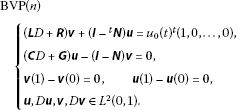In the case of Thomson cable, we do not require $D\mathbit{v}\in {L}^{2}\left(0,1\right)$. By eliminating v, the above equation is rewritten as

$\left\{\begin{array}{c}\left(\mathbit{L}D+\mathbit{R}\right){\left(\mathbit{I}-\mathbit{N}\right)}^{-1}\left(\mathbit{C}D+\mathbit{G}\right)\mathbit{u}+\left(\mathbit{I}{-}^{t}\mathbit{N}\right)\mathbit{u}={u}_{0}{\left(t\right)}^{t}\left(1,0,\dots ,0\right)\phantom{\rule{1em}{0ex}}\left(0

From now on, we put

${u}_{0}\left(t\right)=\left\{\begin{array}{cc}\left({\prod }_{i=1}^{n}\left({L}_{i}{C}_{i}\right)\right)f\left(t\right)\hfill & \left(\text{HC}\right),\hfill \\ \left({\prod }_{i=1}^{n}\left({R}_{i}{C}_{i}\right)\right)f\left(t\right)\hfill & \left(\text{TC}\right).\hfill \end{array}$

By Fourier transform, we have the following matrix equation: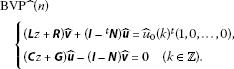We use the abbreviation $z=\sqrt{-1}{\omega }_{k}$. The above equation is rewritten as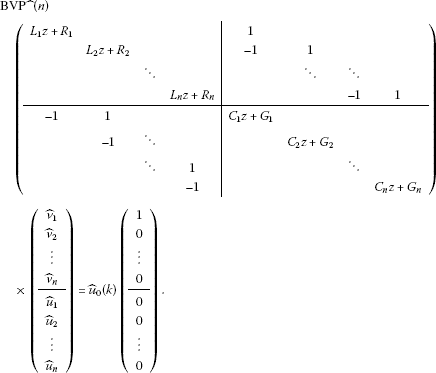(6.1)

By induction, it is easy to see that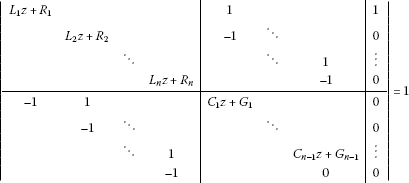holds. The characteristic polynomial is given by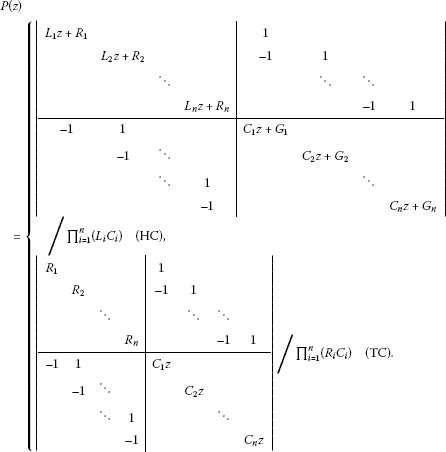Solving the linear equation (6.1) with respect to ${\stackrel{ˆ}{u}}_{n}\left(k\right)$ applying Cramer’s formula, we have

${\stackrel{ˆ}{u}}_{n}\left(k\right)=P{\left(z\right)}^{-1}\stackrel{ˆ}{f}\left(k\right)\phantom{\rule{1em}{0ex}}\left(k\in \mathbb{Z}\right).$

This means that

$P\left(d/dt\right){u}_{n}=f\left(t\right)\phantom{\rule{1em}{0ex}}\left(0

In our previous work , we found that these polynomials (HC) and (TC) are Hurwitz polynomials, in which all roots have positive real parts.

## 7 Examples

Physical meaning of the Sobolev type inequality is that we can estimate the square of maximum of the absolute value of AC output voltage from above by the power of input voltage. The authors believe that this is a new point of view compared with classical theory which mainly considers input-output gain.

In this section, let us calculate the best constants of the Sobolev type inequality for specific cases.

(1 unit Heaviside cable)

The circuit parameters are considered as ${L}_{1}=L$, ${R}_{1}=R$, ${C}_{1}=C$, and ${G}_{1}=G$. The characteristic polynomial is calculated as

$P\left(z\right)=|\begin{array}{cc}Lz+R& 1\\ -1& Cz+G\end{array}|/\left(LC\right)={p}_{0}{z}^{2}+{p}_{1}z+{p}_{2},$

where

${p}_{0}=1,\phantom{\rule{2em}{0ex}}{p}_{1}=\frac{LG+RC}{LC},\phantom{\rule{2em}{0ex}}{p}_{2}=\frac{RG+1}{LC}.$

We impose the constraint ${p}_{1}>2\sqrt{{p}_{2}}$ to have real eigenvalues. Constants ${a}_{0}$ and ${a}_{1}$ are expressed by circuit constants as

$\begin{array}{rcl}{a}_{0}& =& \frac{{p}_{1}}{2}-\sqrt{\frac{{p}_{1}^{2}}{4}-{p}_{2}}\\ =& \frac{LG+RC}{2LC}-\sqrt{\frac{1}{4}{\left(\frac{LG+RC}{LC}\right)}^{2}-\frac{RC+1}{LC}},\\ {a}_{1}& =& \frac{{p}_{1}}{2}+\sqrt{\frac{{p}_{1}^{2}}{4}-{p}_{2}}\\ =& \frac{LG+RC}{2LC}+\sqrt{\frac{1}{4}{\left(\frac{LG+RC}{LC}\right)}^{2}-\frac{RC+1}{LC}}.\end{array}$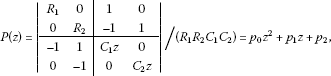where

${p}_{0}=1,\phantom{\rule{2em}{0ex}}{p}_{1}=\frac{1}{{R}_{1}{C}_{1}}+\frac{1}{{R}_{2}{C}_{1}}+\frac{1}{{R}_{2}{C}_{2}},\phantom{\rule{2em}{0ex}}{p}_{2}=\frac{1}{{R}_{1}{R}_{2}{C}_{1}{C}_{2}}.$

We impose the constraint ${p}_{1}>2\sqrt{{p}_{2}}$ to have real eigenvalues. Constants ${a}_{0}$ and ${a}_{1}$ are expressed by circuit constants as

$\begin{array}{rcl}{a}_{0}& =& \frac{{p}_{1}}{2}-\sqrt{\frac{{p}_{1}^{2}}{4}-{p}_{2}}\\ =& \frac{1}{2}\left(\frac{1}{{R}_{1}{C}_{1}}+\frac{1}{{R}_{2}{C}_{1}}+\frac{1}{{R}_{2}{C}_{2}}\right)-\sqrt{\frac{1}{4}{\left(\frac{1}{{R}_{1}{C}_{1}}+\frac{1}{{R}_{2}{C}_{1}}+\frac{1}{{R}_{2}{C}_{2}}\right)}^{2}-\frac{1}{{R}_{1}{R}_{2}{C}_{1}{C}_{2}}},\\ {a}_{1}& =& \frac{{p}_{1}}{2}+\sqrt{\frac{{p}_{1}^{2}}{4}-{p}_{2}}\\ =& \frac{1}{2}\left(\frac{1}{{R}_{1}{C}_{1}}+\frac{1}{{R}_{2}{C}_{1}}+\frac{1}{{R}_{2}{C}_{2}}\right)+\sqrt{\frac{1}{4}{\left(\frac{1}{{R}_{1}{C}_{1}}+\frac{1}{{R}_{2}{C}_{1}}+\frac{1}{{R}_{2}{C}_{2}}\right)}^{2}-\frac{1}{{R}_{1}{R}_{2}{C}_{1}{C}_{2}}}.\end{array}$

For the above two cases, constants ${a}_{0}$ and ${a}_{1}$ satisfy the assumption ${a}_{0}<{a}_{1}$. In both cases, the best constant and best function are computed as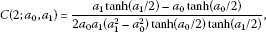(7.1)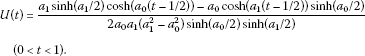(7.2)

Next we consider the case ${p}_{1}=2\sqrt{{p}_{2}}$, where we have multiple root (a general case is shown in the same way). Taking the limit as ${a}_{1}\to {a}_{0}$ in (7.1) and (7.2), we have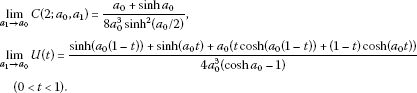## References

1. Kamke E: Differentialgleichungen, Lösungsmethoden und Lösungen I. 3rd edition. Chelsea, New York; 1971.

2. Heaviside O: Electromagnetic Induction and Its Propagation - XLVII. The Electrician, London; 1887:189-191.

3. Nahin PJ: Oliver Heaviside, Sage in Solitude: The Life, Work, and Times of an Electrical Genius of the Victorian Age. IEEE Press, New York; 1988:230-232.

4. Kametaka Y, Nagai A, Watanabe K, Takemura K, Yamagishi H: Giambelli’s formula and the best constant of Sobolev inequality in one dimensional Euclidean space. Sci. Math. Jpn. 2010, 71(1):27-41. e-2009, 621-635

5. Kametaka Y, Takemura K, Yamagishi H, Nagai A, Watanabe K: Heaviside cable, Thomson cable and the best constant of a Sobolev-type inequality. Sci. Math. Jpn. 2008, 68(1):63-79. e-2007, 739-755

6. Neagoe V-E: Inversion of the Van der Monde matrix. IEEE Signal Process. Lett. 1996, 3(4):119-120.

## Acknowledgements

The authors KT and AN are supported by J. S. P. S. Grant-in-Aid for Scientific Research (C) No. 21540148 and No. 20540138.

## Author information

Authors

### Corresponding author

Correspondence to Kazuo Takemura.

### Competing interests

The authors declare that they have no competing interests.

### Authors’ contributions

YK wrote the first draft, KT, KW, AN and HY corrected and improved it and KT prepared the final version. All authors read and approved the final manuscript.

## Authors’ original submitted files for images

Below are the links to the authors’ original submitted files for images.

## Rights and permissions

Reprints and Permissions

Takemura, K., Kametaka, Y., Watanabe, K. et al. Sobolev type inequalities of time-periodic boundary value problems for Heaviside and Thomson cables. Bound Value Probl 2012, 95 (2012). https://doi.org/10.1186/1687-2770-2012-95

• Accepted:

• Published:

• DOI: https://doi.org/10.1186/1687-2770-2012-95

### Keywords

• Hurwitz polynomial
• Sobolev inequality
• best constant
• Green function